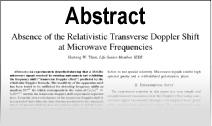Enter the content which will be displayed in sticky bar

# AbstractThe Electrodynamic Origin of the Force of Inertia

Year: 2006
The force of inertial is shown to be a statistical residual force due to the acceleration terms in the derived universal electrodynamic force between vibrating neutral dipoles consisting of atomic electrons vibrating with respect to protons in the nucleus of neutral atoms. The inertial mass is derived and shown to be equal to the gravitational mass. The vibrational mechanism for both gravitational and inertial mass causes the magnitude of both masses to decay over time. The inertial force has a non-radial term , which causes certain observed non-Newtonian inertial gyroscopic motions that will be demonstrated. Arguments will be given that this derived law of inertia is superior to Newton\'s Law of Inertia.